Courses

# Test: Airport Engineering - 3

## 20 Questions MCQ Test Transportation Engineering | Test: Airport Engineering - 3

Description
This mock test of Test: Airport Engineering - 3 for Civil Engineering (CE) helps you for every Civil Engineering (CE) entrance exam. This contains 20 Multiple Choice Questions for Civil Engineering (CE) Test: Airport Engineering - 3 (mcq) to study with solutions a complete question bank. The solved questions answers in this Test: Airport Engineering - 3 quiz give you a good mix of easy questions and tough questions. Civil Engineering (CE) students definitely take this Test: Airport Engineering - 3 exercise for a better result in the exam. You can find other Test: Airport Engineering - 3 extra questions, long questions & short questions for Civil Engineering (CE) on EduRev as well by searching above.
QUESTION: 1

Solution:
QUESTION: 2

### According to ICAO recommendations, what is the rate of elevation correction "for the runway length above MSL?

Solution:

7% per 300m rise in elevation above MSL

QUESTION: 3

### What does the Wind Rose Diagram (WRD) for orientation of airport runway give?

Solution:

Type-I wind rose diagram shows direction and duration of wind. Type-ll wind rose diagram shows direction, duration and intensity of wind,

QUESTION: 4

Which one of the following statements is correct? Compared to IFR conditions, the runaway capacity of a runway operating under VFR conditions:

Solution:

Under the conditions of Visual Flight Rules (VFR), the single runway can handle about 45 to 60 operations per hour. For Instrument Flight Rules (IFR), the capacity of single runway is reduced to about 20 to 40 operations per hours.

QUESTION: 5

As per ICAO recommendation, minimum width of safety area for instrumental runway should be

Solution:
QUESTION: 6

As per ICAO for A, B and C type of airports, maximum effective, transverse and longitudinal grades in percentage respectively are

Solution:
QUESTION: 7

The capacity of a gate which is available to all classes of aircraft as per details given in table will be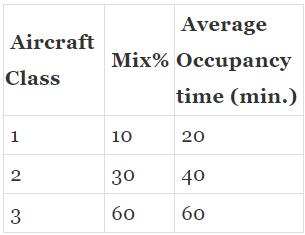Solution:

Since each gate is available for all air-crafts. The gate capacity for a single gate is given by,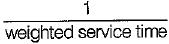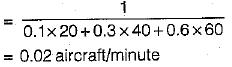QUESTION: 8

The capacity of parallel runway pattern depends upon

Solution:
QUESTION: 9

If the monthly mean of average daily temperature for the hottest month of the year is 25°C and the monthly mean.of the maximum daily temperature of the same month of the year is 46ºC, the airport reference temperature is

Solution:

This is given by,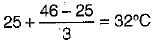QUESTION: 10

Consider the following statements regarding ICAO recommendation for correction to basic runway length
1. The basic runway length should be increased at the rate of 7 percent per 300 m rise in elevation above the mean sea level.
2. The basic runway length after having been corrected for elevation should be further increased at the rate of 1 percent for every 1°C rise in airport reference temperature above the standard atmospheric temperature at that elevation.
3. The runway length after having been corrected for elevation and temperature should be further 1 percent of effective gradient.
Which of these statements is/are correct? .

Solution:

ICAO does not recommend any specific correction for the gradient. Correction for gradient in (c) is as per FAA recommendation.

QUESTION: 11

The total length of a runway is 1000 m. The elevation at distance, 0, 200 m, 400 m, 600 m, 800 m and 1000 m are 100.0 m, 101.0 m respectively. The effective gradient of runway will be

Solution:

Effective gradient of runway is defined as the maximum difference in elevation between the highest and lowest points on runway divided by total length of runway. So it is given by,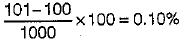QUESTION: 12

The length of runway under standard conditions is 2000 m. The elevation of airport site is 300 m. Its reference temperature is 33.05°C. if the runway is to be constructed with an effective gradient of 0.25 percent, the correct runway length will be

Solution:

Correction for elevation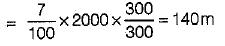Corrected length
= 2000 + 140 = 2140 m
Standard atmospheric temp at given elevation
= 15 - 0.0065 x 300 = 13.05°C
Correction for temperature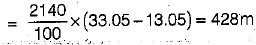Corrected.Jength = 2140 + 428 = 2568 m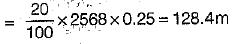Corrected length
= 2568 + 128.4 = 2696.4 m = 2700 m

QUESTION: 13

As per ICAO, the minimum basic runway length for A and E type of airport will be

Solution:

The minimum basic length for B, C and D types of airport are 1500 m, 900 m and 750 m respectively.

QUESTION: 14

Zero fuel weight of an aircraft is

Solution:
QUESTION: 15

The cruising speed for the aircraft is 500 kmph. If there is a head wind of 50 kmph, then the air speed and ground speed of the aircraft respectively will be

Solution:

Ground speed is same as cruising speed and it is the speed of aircraft wrt ground. Air speed is the speed of aircraft relative to wind.

QUESTION: 16

Calm period is the percentage of time during which wind intensity is less than

Solution:
QUESTION: 17

For determining the basic runway length, the landing case requires that aircraft should come to a stop within p% of the landing distance. The value of p is

Solution:
QUESTION: 18

According to ICAO, all markings on the runways are

Solution:

Ail marking on taxiway are yellow.

QUESTION: 19

The width and interval of transverse centre line bars along the extended centre line of runway, in approach lighting system are

Solution:
QUESTION: 20

In Instrumental landing system, the middle markers are located

Solution: